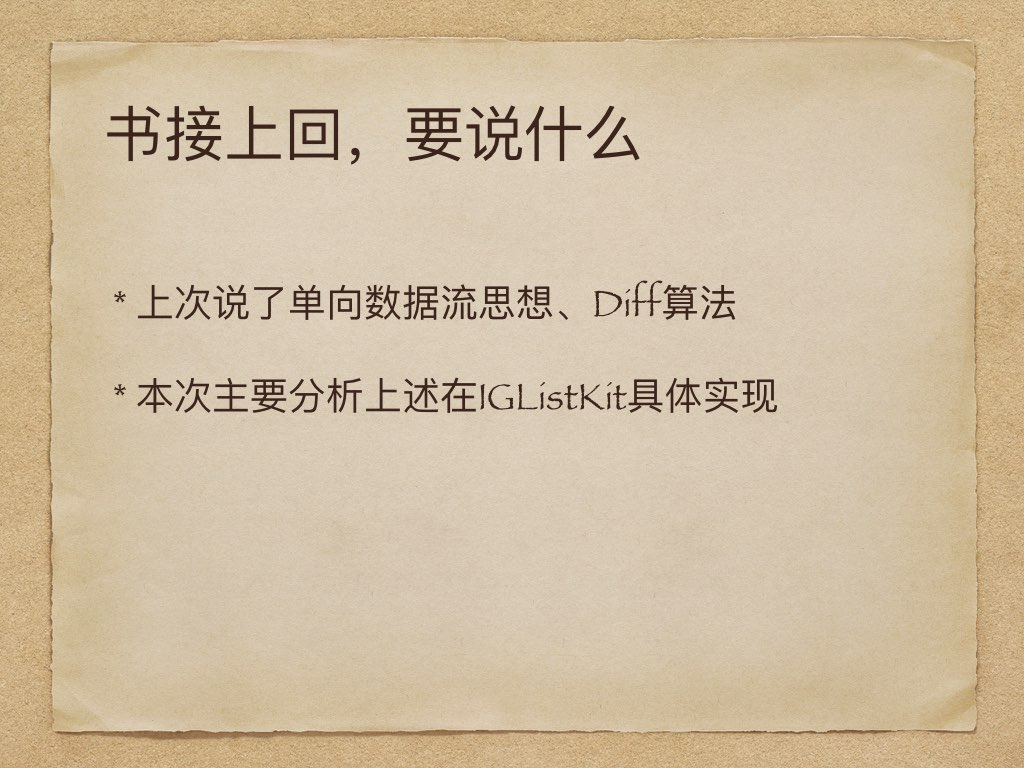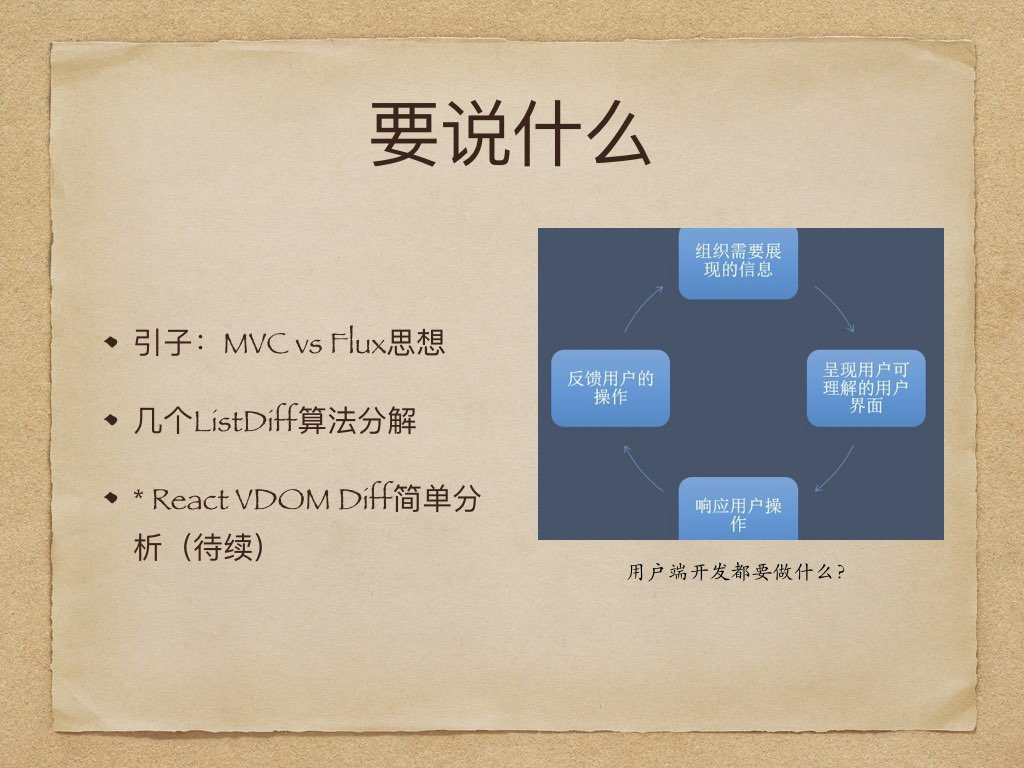# 面试常见：iOS Category

Read more   2018/08/09 posted in  技术

# 【IGListKit】学习与分析2Read more   2018/04/16 posted in  技术

# 【IGListKit】ListDiff 学习与分析1Read more   2018/04/02 posted in  技术

# 【iOS】从同步锁到多线程

## 一、几种线程同步方式

### 1、自旋锁 `OSSpinLock`

``````__block OSSpinLock oslock = OS_SPINLOCK_INIT;

//线程2
dispatch_async(dispatch_get_global_queue(DISPATCH_QUEUE_PRIORITY_HIGH, 0), ^{
NSLog(@"线程2 准备上锁");
OSSpinLockLock(&oslock);
NSLog(@"线程2");
OSSpinLockUnlock(&oslock);
NSLog(@"线程2 解锁成功");
});
//线程1
dispatch_async(dispatch_get_global_queue(DISPATCH_QUEUE_PRIORITY_BACKGROUND, 0), ^{
NSLog(@"线程1 准备上锁");
OSSpinLockLock(&oslock);
NSLog(@"线程1 sleep");
sleep(4);
NSLog(@"线程1");
OSSpinLockUnlock(&oslock);
NSLog(@"线程1 解锁成功");
});
``````

### 2、信号量 `dispatch_semaphore`

``````dispatch_semaphore_t signal = dispatch_semaphore_create(1);
dispatch_time_t timeout = dispatch_time(DISPATCH_TIME_NOW, 3.0f * NSEC_PER_SEC);

//线程1
dispatch_async(dispatch_get_global_queue(DISPATCH_QUEUE_PRIORITY_DEFAULT, 0), ^{
NSLog(@"线程1 等待ing");
dispatch_semaphore_wait(signal, timeout); //signal 值 -1
NSLog(@"线程1 sleep");
sleep(2);
NSLog(@"线程1");
dispatch_semaphore_signal(signal); //signal 值 +1
NSLog(@"线程1 发送信号");
});

//线程2
dispatch_async(dispatch_get_global_queue(DISPATCH_QUEUE_PRIORITY_DEFAULT, 0), ^{
NSLog(@"线程2 等待ing");
dispatch_semaphore_wait(signal, timeout);
NSLog(@"线程2 sleep");
sleep(2);
NSLog(@"线程2");
dispatch_semaphore_signal(signal);
NSLog(@"线程2 发送信号");
});
``````

dispatch_semaphore_wait中传入的timeout表示最长加锁时间，此处sleep如果为4，则在3s后会自动释放锁，其它线程可以获取信号并继续运行。

semaphore ['sɛməfɔr] ： 信号；旗语；

### 3、互斥锁 `pthread_mutex`

``````static pthread_mutex_t pLock;
pthread_mutex_init(&pLock, NULL);

//1.线程2
dispatch_async(dispatch_get_global_queue(DISPATCH_QUEUE_PRIORITY_HIGH, 0), ^{
NSLog(@"线程2 准备上锁");
pthread_mutex_lock(&pLock);
NSLog(@"线程2");
pthread_mutex_unlock(&pLock);
});

//2.线程1
dispatch_async(dispatch_get_global_queue(DISPATCH_QUEUE_PRIORITY_DEFAULT, 0), ^{
NSLog(@"线程1 准备上锁");
pthread_mutex_lock(&pLock);
sleep(3);
NSLog(@"线程1");
pthread_mutex_unlock(&pLock);
});
``````

pthread 表示 POSIX thread，定义了一组跨平台的线程相关的 API，pthread_mutex 表示互斥锁。

#### `pthread_mutex(recursive)` 递归锁

``````static pthread_mutex_t pLock;
pthread_mutexattr_t attr;
pthread_mutexattr_init(&attr); //初始化attr并且给它赋予默认
pthread_mutexattr_settype(&attr, PTHREAD_MUTEX_RECURSIVE); //设置锁类型，这边是设置为递归锁
pthread_mutex_init(&pLock, &attr);
pthread_mutexattr_destroy(&attr); //销毁一个属性对象，在重新进行初始化之前该结构不能重新使用

//1.线程1
dispatch_async(dispatch_get_global_queue(DISPATCH_QUEUE_PRIORITY_DEFAULT, 0), ^{
static void (^RecursiveBlock)(int);
RecursiveBlock = ^(int value) {
pthread_mutex_lock(&pLock);
if (value > 0) {
NSLog(@"value: %d", value);
RecursiveBlock(value - 1);
}

};
NSLog(@"线程1 准备上锁");
RecursiveBlock(5);
NSLog(@"线程1");
pthread_mutex_unlock(&pLock);
NSLog(@"线程1 解锁");
});

//2.线程2
dispatch_async(dispatch_get_global_queue(DISPATCH_QUEUE_PRIORITY_DEFAULT, 0), ^{
NSLog(@"线程2 准备上锁");
pthread_mutex_lock(&pLock);
NSLog(@"线程2");
pthread_mutex_unlock(&pLock);
NSLog(@"线程2 解锁");
});
``````

### 4&5、NS前缀的两个锁`NSLock`、`NSRecursiveLock`

``````NSLock *lock = [NSLock new];
[lock lock];
NSLog(@"加锁运行");
[lock unlock];

NSRecursiveLock *recursiveLock = [NSRecursiveLock new];
[recursiveLock lock];
NSLog(@"加锁运行");
[recursiveLock unlock];
``````

NS开头的类都是对CoreFoundation的封装，只是易用一些。NSRecursiveLock为递归锁，可以在循环和递归中使用。

NSLock 只是在内部封装了一个 pthread_mutex，属性为 PTHREAD_MUTEX_ERRORCHECK，它会损失一定性能换来错误提示。理论上 NSLock 和 pthread_mutex 拥有相同的运行效率，实际由于封装的原因，会略慢一点，由于有缓存存在，相差不会很多，属于相同数量级。
NSRecursiveLock 与 NSLock 的区别在于内部封装的 pthread_mutex_t 对象的类型不同，NSRecursiveLock 的类型为 PTHREAD_MUTEX_RECURSIVE。

### 7、条件锁 `NSConditionLock`

``````NSConditionLock *cLock = [[NSConditionLock alloc] initWithCondition:0];

//线程2
dispatch_async(dispatch_get_global_queue(DISPATCH_QUEUE_PRIORITY_HIGH, 0), ^{
[cLock lockWhenCondition:1];
NSLog(@"线程2");
[cLock unlockWithCondition:3];
});
//线程1
dispatch_async(dispatch_get_global_queue(DISPATCH_QUEUE_PRIORITY_LOW, 0), ^{
if([cLock tryLockWhenCondition:0]){
NSLog(@"线程1");
[cLock unlockWithCondition:1];
}else{
NSLog(@"失败");
}
});

//线程3
dispatch_async(dispatch_get_global_queue(DISPATCH_QUEUE_PRIORITY_DEFAULT, 0), ^{
[cLock lockWhenCondition:3];
NSLog(@"线程3");
[cLock unlockWithCondition:2];
});
``````

### 8、简单易用的条件锁 `@synchronized`

``````@synchronized (self) {
NSLog(@"加锁运行");
}
``````

@synchronized 实际上是把修饰对象当做锁来使用。这是通过一个哈希表来实现的，OC 在底层使用了一个互斥锁的数组(你可以理解为锁池)，通过对对象去哈希值来得到对应的互斥锁。

## 二、5S下测试一千万次加锁解锁时间测试``````#define ITERATIONS (10000000) // 1千万
+ (void)test
{
double then, now;

@autoreleasepool {

// 普通锁 NSLock
NSLock *lock = [NSLock new];
then = CFAbsoluteTimeGetCurrent();
for(NSInteger i = 0; i < ITERATIONS; ++i)
{
[lock lock];
[lock unlock];
}
now = CFAbsoluteTimeGetCurrent();
NSLog(@"NSLock: %f sec\n", now-then);

// 互斥锁 pthread_mutex
pthread_mutex_t mutex = PTHREAD_MUTEX_INITIALIZER;
then = CFAbsoluteTimeGetCurrent();
for(NSInteger i = 0; i < ITERATIONS; ++i)
{
pthread_mutex_lock(&mutex);
pthread_mutex_unlock(&mutex);
}
now = CFAbsoluteTimeGetCurrent();
NSLog(@"pthread_mutex: %f sec\n", now-then);

// 递归锁 pthread_mutex(recursive)
static pthread_mutex_t pLock;
pthread_mutexattr_t attr;
pthread_mutexattr_init(&attr); //初始化attr并且给它赋予默认
pthread_mutexattr_settype(&attr, PTHREAD_MUTEX_RECURSIVE); //设置锁类型，这边是设置为递归锁
pthread_mutex_init(&pLock, &attr);
pthread_mutexattr_destroy(&attr); //销毁一个属性对象，在重新进行初始化之前该结构不能重新使用

then = CFAbsoluteTimeGetCurrent();
for(NSInteger i = 0; i < ITERATIONS; ++i)
{
pthread_mutex_lock(&pLock);
pthread_mutex_unlock(&pLock);
}
now = CFAbsoluteTimeGetCurrent();
NSLog(@"pthread_mutex(recursive): %f sec\n", now-then);

// 自旋锁 OSSpinlock
OSSpinLock spinlock = OS_SPINLOCK_INIT;
then = CFAbsoluteTimeGetCurrent();
for(NSInteger i = 0; i < ITERATIONS; ++i)
{
OSSpinLockLock(&spinlock);
OSSpinLockUnlock(&spinlock);
}
now = CFAbsoluteTimeGetCurrent();
NSLog(@"OSSpinlock: %f sec\n", now-then);

// synchronized
id obj = [NSObject new];
then = CFAbsoluteTimeGetCurrent();
for(NSInteger i = 0; i < ITERATIONS; ++i)
{
@synchronized(obj)
{
}
}
now = CFAbsoluteTimeGetCurrent();
NSLog(@"@synchronized: %f sec\n", now-then);

// dispatch_semaphore
dispatch_semaphore_t lockSemaphore = dispatch_semaphore_create(1);
then = CFAbsoluteTimeGetCurrent();
for(NSInteger i = 0; i < ITERATIONS; ++i)
{
dispatch_semaphore_wait(lockSemaphore, DISPATCH_TIME_FOREVER);
dispatch_semaphore_signal(lockSemaphore);
}
now = CFAbsoluteTimeGetCurrent();
NSLog(@"dispatch_semaphore: %f sec\n", now-then);

NSCondition *cLock = [NSCondition new];
then = CFAbsoluteTimeGetCurrent();
for(NSInteger i = 0; i < ITERATIONS; ++i)
{
[cLock lock];
[cLock unlock];
}
now = CFAbsoluteTimeGetCurrent();
NSLog(@"NSCondition: %f sec\n", now-then);

NSRecursiveLock *rLock = [NSRecursiveLock new];
then = CFAbsoluteTimeGetCurrent();
for(NSInteger i = 0; i < ITERATIONS; ++i)
{
[rLock lock];
[rLock unlock];
}
now = CFAbsoluteTimeGetCurrent();
NSLog(@"NSRecursiveLock: %f sec\n", now-then);

// dispatch_barrier_async
dispatch_queue_t queue = dispatch_queue_create("xyz.chaisong.lock", DISPATCH_QUEUE_SERIAL);
then = CFAbsoluteTimeGetCurrent();
for(NSInteger i = 0; i < ITERATIONS; ++i)
{
dispatch_barrier_async(queue, ^{
});
}
now = CFAbsoluteTimeGetCurrent();
NSLog(@"dispatch_barrier_async: %f sec\n", now-then);
}
}
``````

OSpinlock为什么效率奇高主要原因是：并没有进入系统kernel，使用它可以节省系统调用和上下文切换。

## 三、一些多线程的基础知识

### 3、自旋锁和互斥锁

#### 自旋锁实现原理

``````lock = 0;
do{
while(test_and_set(&lock));
临界区
lock = 0;
其余部分
} while(1)
``````

test_and_set用来保证条件判断的原子性操作，lock为旗标。

#### 信号量的实现

``````int sem_wait (sem_t *sem) {
int *futex = (int *) sem;
if (atomic_decrement_if_positive (futex) > 0)
return 0;
int err = lll_futex_wait (futex, 0);
return -1;
)
``````

## 四、多线程中的常见术语

• 条件(condition)
一个用来同步资源访问的结构。线程等待某一条件来决定是否被允许继续运行，直到其他线程显式的给该条件发送信号。
• 临界区(critical section)
同一时间只能不被一个线程执行的代码。
• 输入源(input source)
一个线程的异步事件源。输入源可以是基于端口的或手工触发，并且必须被附加到某一个线程的run loop上面。
• 可连接的线程(join thread)
退出时资源不会被立即回收的线程。可连接的线程在资源被回收之前必须被显式脱离或由其他线程连接。可连接线程提供了一个返回值给连接它的线程。
• 主线程(main thread)
当创建进程时一起创建的特定类型的线程。当程序的主线程退出，则程序即退出。
• 互斥锁(mutex)
提供共享资源互斥访问的锁。一个互斥锁同一时间只能被一个线程拥有。试图获取一个已经被其他线程拥有的互斥锁，会把当前线程置于休眠状态知道该锁被其他线程释放并让当前线程获得。
• 操作对象(operation object)
NSOperation类的实例。操作对象封装了和某一任务相关的代码和数据到一个执行单元里面。
• 操作队列(operation queue)
NSOperationQueue类的实例。操作队列管理操作对象的执行。
• 进程(process)
应用或程序的运行时实例。一个进程拥有独立于分配给其他程序的的内存空间和系统资源（包括端口权限）。进程总是包含至少一个线程（即主线程）和任意数量的额外线程。
• 递归锁(recursive lock)
可以被同一线程多次锁住的锁。
• 信号量(semaphore)
一个受保护的变量，它限制共享资源的访问。互斥锁(mutexes)和条件(conditions)都是不同类型的信号量。
• 任务(task)
要执行的工作数量。尽管一些技术(最显著的是Carbon 多进程服务—Carbon Multiprocessing Services)使用该术语的意义有时不同，但是最通用的用法是表明需要执行的工作数量的抽象概念。
• 线程(thread)
进程里面的一个执行过程流。每个线程都有它自己的栈空间，但除此之外同一进程的其他线程共享内存。
2017/02/06 posted in  技术